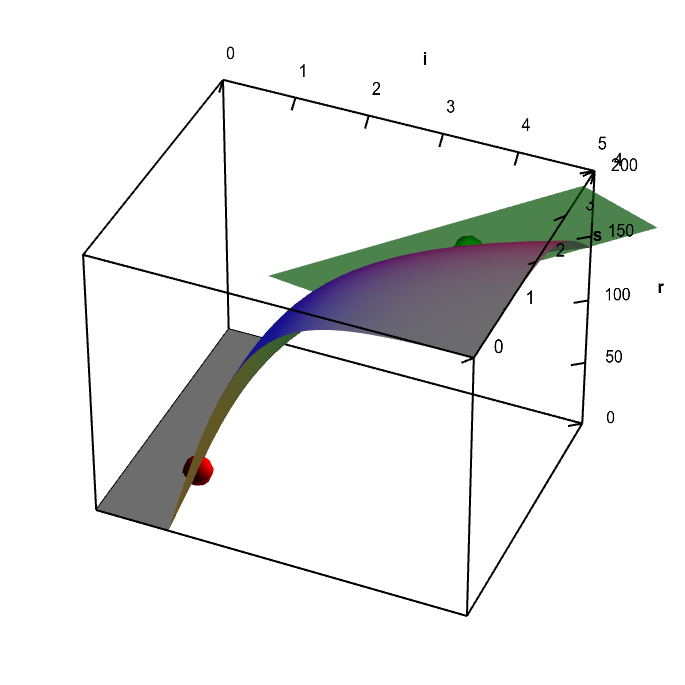# Math Insight

### Applet: Neuron firing rate function with tangent planeA fictitious representation of the firing rate $r(i,s)$ of a neuron in response to an input $i$ and nicotine level $s$. The graph of the function has a tangent plane at the location of the green point, so the function is differentiable there. By rotating the graph, you can see how the tangent plane touches the surface at the that point. You can move the green point anywhere on the surface; as long as it is not along the fold of the graph (where the red point in constrained to be), you can see the tangent plane showing that the function is differentiable. There is no tangent plane to the graph at any point along the fold of the graph (you can move the red point to any point along this fold). The function $r(i,s)$ is not differentiable at any point along the fold. As further evidence of this non-differentiability, the tangent plane jumps to a different angle when you move the green point across the fold.